# A Weight Based Feature Extraction Model on Multifaceted Multimedia Bigdata Using Convolutional Neural Network

A Weight Based Feature Extraction Model on Multifaceted Multimedia Bigdata Using Convolutional Neural Network
Department of Computer Science and Engineering, Annamalai University, Chidambaram 608002, Tamil Nadu, India

Department of Information of Technology, Vignan's Nirula Institute of Technology & Science for Women, Guntur 522009, Andhra Pradesh, India

Corresponding Author Email:
venkatresearchau@gmail.com
Page:
729-735
|
DOI:
https://doi.org/10.18280/isi.250603
2 October 2020
|
Accepted:
5 December 2020
|
Published:
31 December 2020
| Citation

OPEN ACCESS

Abstract:

This paper intends to present main technique for feature extraction on multimeda getting well versed and a challenging task to handle big data. Analyzing and feature extracting valuable data from high dimensional dataset challenges the bounds of measurable methods and strategies. Conventional techniques in general have less performance while managing high dimensional datasets. Lower test size has consistently been an issue in measurable tests, which get bothered in high dimensional information due to more equivalent or higher component size than the quantity of tests. The intensity of any measurable test is legitimately relative to its capacity to lesser an invalid theory, and test size is a significant central factor in producing probabilities of errors for making substantial ends. Thus one of the effective methods for taking care of high dimensional datasets is by lessening its measurement through feature selection and extraction with the goal that substantial accurate data can be practically performed. Clustering is the act of finding hidden or comparable data in information. It is one of the most widely recognized techniques for realizing useful features where a weight is given to each feature without predefining the various classes. In any feature selection and extraction procedures, the three main considerations of concern are measurable exactness, model interpretability and computational multifaceted nature. For any classification model, it is important to ensure that the productivity of any of these three components isn't undermined. In this manuscript, a Weight Based Feature Extraction Model on Multifaceted Multimedia Big Data (WbFEM-MMB) is proposed which extracts useful features from videos. The feature extraction strategies utilize features from the discrete cosine methods and the features are extracted using a pre-prepared Convolutional Neural Network (CNN). The proposed method is compared with traditional methods and the results show that the proposed method exhibits better performance and accuracy in extracting features from multifaceted multimedia data.

Keywords:

multifaceted multimedia data, feature extraction, feature selection, classification, video object detection, convolutional neural network

1. Introduction

Information characterization, featured determination and extraction are the significant research zones in the field of information mining. Information arrangement issues center around learning a lot of organized information, and later use data from preparing information to anticipate the idea of any new data point. Information classification can be directed with unsupervised learning strategy which depends on the accessibility of data on preparing information. Administered information, classification and calculation contemplates preparing and create a grouping model to characterize any arrangement of new information. Unsupervised calculations then again attempt to discover designs in the unlabeled preparing information.

The multimedia analysis more help for large, complex collections system to navigate multifaceted classification. A faceted search system that combines facets from text, image and video stream. In image features are constructing based on low-level or deep visual features of the images. The facets mean for filtering or pivoting combining collections. The data set can be searched using facets fundamental on aggregations of raw extracted features. Feature extraction is useful for removing irrelevant or redundant data reducing feature vectors and dimensionality.

The feature grouping is that information likeness is sufficient to portray reduced classes in a component space with no other sort of data accessible. By and by, the direction absolutely by information renders the clustering procedure safe to characteristics of the information and the information taking care of plan, and results in exceptional increment of multifaceted nature with the information size as the issue isn't NP hard. Creating sensibly with great outcomes, a proficient procedure is effectively joined with different strategies in bigger frameworks focusing on consequently dividing the whole informational index into a set number of multiple gatherings.

The data separated from the procedure of broad information mining significantly reduces computational expenses and in way it expands the adequacy and effectiveness. It turns out to be computationally conceivable in finding the model information from huge measured unstructured information likewise by utilizing fitting mining techniques. The extraction of certain, possibly valuable and already obscure data from information in a nontrivial way is for the most part alluded to as information mining. It may be well characterized completely as the strategy for drawing helpful data from an enormous multimedia database. The task of the information mining is limited to information revelation from database for extraction of useful features from the video given as input.

Feature extraction is used for extracting relevant important features in computerized images in combination with clustering. Feature extraction could reveal coordinates among various regions in an image which demonstrates the proximity of features. The method of reasoning behind utilizing clustering is that we recognize unique matches and features for example coordination among unique regions of an image. The vast majority of clustering calculations produce the best groups implying that each pixel group has particularly a place with one cluster. In any case, some datasets have been characteristically covering data which could be clarified by covering grouping techniques.

The CNN is mainly used for extracting hidden features in images for accurate object detections in the image or in a video. The structure of CNN representing hidden layers is depicted in Figure 1 The objects in the video is clearly identified by extracting normal pixels and hidden features.

A Convolutional Neural Network is representing of number of inputs and an output layer, it consists as more hidden layers. The input like as number of images and videos. Every convolutional data can be used to train feature then classify the data. The initial purpose of convolution to extract from the input image with hidden system. CNN is representing the weights among the input and hidden layer with recursively at nearby time levels.

A Convolutional neural system comprises of neurons in different layers; the info layer such as the resultant layer and at least one hidden layer. Systems with at least two hidden layers are called deep neural systems. The information layer comprises of an information and the result layer comprises of a worth showing whether the neuron is initiated or not. On account of classification of pixels from an image, the neurons in the output layer speak to the various classes. Every one of the neurons in the output layer brings about a delicate max esteem which portrays the likelihood of the information having a place with that class.

## 1.png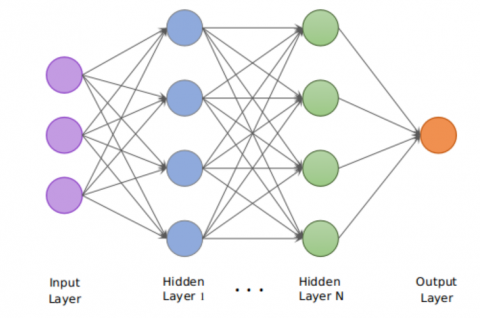Figure 1. Typical architecture of fully-connected Convolutional Neural Network

The contribution to a neuron is the weighted outputs of the neurons in the hidden layer a layer is completely associated, it comprises of the output from all neurons in the hidden layer. The weight controls the measure of impact of the output of a neuron that has on the following neuron. The hidden layers of each includes various combinations of the weighted outputs of the past layers. In the way with expanded number of hidden layers, increasingly complex features can be made. The technique can streamlined be portrayed as making complex mixes out of the data about the information which effectively maps the information the right output. In the preparation part, when the system is prepared, those features are shaped which can be imagined as a grouping model. In the assessment part, that model is utilized characterizing new data. The process of feature extraction using CNN is depicted in Figure 2.

## 2.png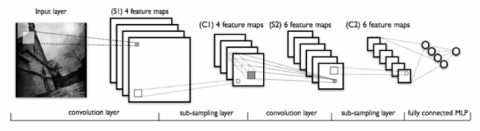Figure 2. Process in convolutional neural network

2. Literature Survey

Ahmadi et al.  have essentially centered in giving better extraction of data from images by improving the nature of the images and by utilizing robotized image handling strategies. To improve the image quality one of the most straightforward and powerful systems is Histogram Equalization (HE). The proposed model which depends on Principal Component Analysis PCA . The model is changed into eigen space and is crossed by the key parts. The dimensionality reduction property of PCA will diminish the size of images and lessen the time required for registering the closeness among features. Analyses were led in utilizing diverse image sizes. The computational time for registering the standardized cross-connection was as low as 0.05 seconds while the other three best in class methods were around 7 seconds.

Another technique which investigated the electric system recurrence in a echo sign to decide credibility or altering . They additionally proposed an upgraded strategy for sound alter location.

The recognizing video outline duplication dependent on the fingerprints that are removed from discrete cosine transformation (DCT) coefficients . In another work done by Zhou et al.  who proposed a novel model for video object identification. The model depended on data combination and Feature selection.

Oramas et al.  investigated the request issues in image mining, progression in image mining. They proposed an information driven framework for image mining. In that they made out four degrees of information such as Pixel level, Object level, semantic thought level, and model and data level. This strategy doesn't well in general great quantity. The structure for mining images by concealing substance. Their structure gives the probability of using evaluation of resemblance among images and two sorts of quantization [7-9]. The procedure here presented ponders just recuperation points. Considerations like multifaceted nature and time execution are not treated here. The considerations presented are only somewhat advanced in feature selection.

Other visual Features, for instance, surface, shape, and use of compacted images can be perceived for additional extension in object detection. Hogenboom et al.  introduced image mining in image recuperation, portrayed another system for image recuperation using irregular state semantic features . It relies upon extraction of low level concealing, shape and surface properties and their change into strange state semantic features using clustering with the help of an image mining technique .

The image composing review in the image updating frameworks for concealing image improvement. The image clearness in image is diminished in view of lighting, atmosphere and image manipulation issues, etc. when the image is spoiled due to commotions and the loss of data in the photos is moreover possible . To recover information from the flawed image, image update methodology was made. Various procedures like Contrast Stretching, Histogram Equalization and its improvement adjustments, Homomorphic Filtering, Retinex, and Wavelet Multiscale Transform are being utilized for improving the quality and then extracting features [14-16].

The image frameworks improvement where the guideline community is given to the point getting ready systems and histogram dealing with the standard inspiration driving image redesign is to process the data image in such a manner, to the point that the output is progressively relevant to the applications . To improve the visual unmistakable quality of the photos there are colossal number choices to renovate frameworks .

Nuray-Turan et al.  presented an assessment in which the web searcher is utilized for recouping a comprehensive number of images using a given substance based request. In a low level image retrieval process, similar image handling work is given to customer to fill in the information request for image likeness depictions. The techniques incorporate wide areas, for example image division, image feature extraction, depiction, mapping of Features to semantics, accumulating and requesting, image similarity estimation for extracting only relevant features for object detection in images [20-22].

3. Proposed Method

Feature extraction is most fundamental procedure for accurate object identification of objects from big data. Features are coordinated into three sorts that are low, middle and high level state. Low level Features are concealing, surface and Middle level part is shape and high level component is semantic of features in images . Hiding relevant features is by far the most generally perceived visual component used on a very basic level taking into account the straightforwardness of isolating hidden information from images. Surface and shape are moreover key sections of human visual perception. Like hiding, this makes it an essential part to consider while addressing image databases. Hiding Features in image recuperation, the hiding an extensively used component. Various methods are utilized to isolate hiding components from images. Here a segment of the procedure is depicted in Figure 3.

In adaptability the clustering strategies, they turned out to become extremely familiar for performing object detection. In the proposed Weight Based Feature Extraction Model on Multifaceted Multimedia Big Data (WbFEM-MMB) the size of the information expanded and a basic use of various leveled classification turned into excessively usefulness. The time irregularity of progressive grouping is where the quantity of Features is extracted. Multifaceted clustering followed various leveled grouping as a method for all the while clustering the Features of a dataset prompting progressively in important groups. It was indicated that this kind of clustering performs better than various leveled clustering with regards to microarray information. However it is yet a computationally requesting strategy. A many number of different strategies have been actualized for separating just the significant data from the microarrays along these lines selected features.

## 3.png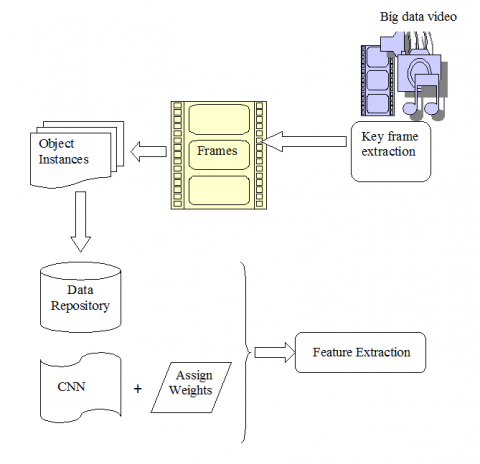Figure 3. Object feature detection from images

Clustering procedure is a mainstream and basic procedure of separating data and relevant pixels from resource without having any earlier information from the items. This might be clarified as the procedure for looking through homogenous Features through pixel values for conceivable feature extraction and clustering. It is important to recognize homogeneity and homogeneous items since the extraordinary estimations of image pixels have been constantly affected by its neighbor pixels. Consequently, the methodology acknowledges that the framework still could isolate comparable pixels in extricating data from video images.

By looking at the dark rationality vectors of images, a proximity measure is proposed. The comparability measure between two image frames X and Y is considered with the parameters:

$I M(\operatorname{diff}(X, Y))=\sum_{j=1}^{n}\left|\alpha_{j}-\alpha_{j}^{\prime}\right|(\mathrm{X})+\left|\beta_{j}-\beta_{j}^{\prime}\right|(\mathrm{Y})$     (1)

where, IM is function for image measure, X and Y values are coordinates for images and n is number of iterations α and β are difference of optimize X and Y coordinates:

$\text { similarity of pixels }=1-\frac{\text { differentiating pixels }}{\text { all pixels } * 2}+\mathrm{X}+\mathrm{Y}$     (2)

The Minkowski distance is the difference between two vectors of equal length (P = Q) of considered images frames in video. It is defined as:

$d_{\min }(a, b)=\left(\sum_{i=1}^{n}\left|x_{i}-y_{i}\right|^{p+q}\right)^{1 / p}$     (3)

where, the minimum distance of order p. p and q are an integers or parameters between two points. xi=(x1, x2,...,xn) and yi= =(y1, y2,...,yn). When p < 1, the distance (0, 0) and (1,1) is 21/p >2.

The time complexity of the Minkowski distance for all p is O(n) and thus determining the distance matrix with this measure takes O(nN2 ) time that is low when compared to existing models.

$d_{e u c}(P, Q)=\sqrt{\sum_{i=1}^{n}\left(a_{i}-b_{i}\right)^{2}}$     (4)

where, Distance complexity of P, Q values and n is number of iterations. In equation a, b values are two clusters ai = (a1, a2,...,an) and bi =(b1, b2,...,bn).

The criterion function in partition clustering method is the squared error criterion considered with isolated and compact clusters. The squared error of a clustering of a pattern set is calculated as

$C(X, Y)=\sum_{j=1}^{k} \sum_{i=1}^{n_{j}}\left|x_{i}^{(j)}-y_{j}\right|^{2}-d_{e u c}(P, Q)$     (5)

where, X and Y values are cluster points and euc mean Euclidian distance for cluster distance. x and y values are equivalent to minimizing squared deviations of pair wise points.

This means that each cluster contains memberships and each of them is characterized by a degree value between 0 and 1. The weights ‘W’ of every pixel ‘p’ extracted is calculated and assigned based on the relevant pixel for object identification and calculating weights is done as

$W(p)=\sum_{i=1}^{N} \sum_{k=1}^{K} u_{i j}\left|x_{i}-c_{k}\right|^{2}+\operatorname{Rand}(C(X, Y))$     (6)

where, $c_{k}=\sum_{i=1}^{N} u_{i k} x_{i}$ is the $k^{t h}$ cluster center of object pixels. From the image, the foreground and back ground pixels are separated and each group of pixels form a unique cluster represented as:

$\delta=\log p\left(\exp ^{-1}\left[\sum_{i=0}^{8}\left(\frac{p_{i}-q_{c}}{2}\right)+W(p)\right]\right)$     (7)

The process of extracting only object features from the image frame is represented with the following algorithm

Input. Image frame from video having k features.

Output. Features that extract object from the image.

Step-1: For each image I ɛ DS(I) the sequence of pixel extractions do

Step-2: Create a cluster set CS(I(X)) with N Samples from the images.

Step-3: Let CS1 ,CS2, …, CS(N) be the N clusters formed with $\left|C S_{m s}\right| \leq\left|C S_{i s}\right|$ for $1 \leq i \leq N$ and $1 \leq \mathrm{I} \leq \mathrm{N}$.

Step-4: Let $W_{I}$  be the weight of $D S(I)_{N}$

Step-5: Foreach f feature from Image I

do

$F(I)_{i}=\left\{X^{\prime} \mid Y^{\prime} \notin C S_{N(i-1)} \text { and } s^{\prime} \varepsilon \operatorname{DS}(\mathrm{I})\right\}$

Weights are calculated for pixels in the object as

$\mathrm{W}\left(\mathrm{p}_{1}, \mathrm{p}_{2}, \ldots, \mathrm{p}_{\mathrm{N}}\right)=\frac{1}{\sum_{\mathrm{i}}^{\mathrm{N}} \mathrm{W}_{\mathrm{i}}} \sum_{\mathrm{i}}^{\mathrm{N}} u_{i j}\left|x_{i}-c_{k}\right|^{2}+\mathrm{v}\left(\mathrm{f}_{\mathrm{i}}\right)+\mathrm{k}$

Compute the first order Derivative sin$\left(\frac{d v_{i}}{d x}\right)$

Compute matrix of distance $\mathrm{D}(\mathrm{i}, \mathrm{j})=\mathrm{d}\left(v_{i}, v_{j}\right)$; I,j=1..n

Clustering:

Cluster set CS(i) = N div $\varepsilon$ CS

$\varepsilon$: number of preselected features

N: initial number of features

While N>1

D(P) = sort W(I)

Cluster $G_{k}$  = select the i $\in$ first features from D(P)

N = N- $C S_{N(i-1)} \in D S(I n)$

Update CS

Step-6: For each pixel $p^{\mathrm{i}} \in I(N i)_{N}$ do

if $\mathrm{W}\left(\mathrm{pV}^{\mathrm{i}}\right)$ is greater than $\mathrm{W}\left(\mathrm{qV}^{\mathrm{i}}\right)$ then send into cluster CS(p) and recompute $\mathrm{W}((\mathrm{p}+1) \mathrm{Vi})$

The angle ‘θ’ is given by the principal value in the range (−π, π) of the inverse tangent function of the slope of the line connecting the two points for fixing the pixels in a particular angle. The pixel rotation is done as:

$\theta=\arctan \left(\frac{y_{2}-y_{1}}{x_{2}-x_{1}}\right)$     (8)

The clusters generated are maintained as each cluster of object features are considered in step by step and then they are considered for object detection. The clusters are grouped as

$C G(C S)=\sum_{i=1}^{N} \sum_{j=i+1}^{N} d_{i j}^{2}+\operatorname{Rand}(C(X, Y))+L$     (9)

where, CG is clusters are grouped and CS clusters. N is the total clusters, L is the constant. An edge detector of the object was used in the image before applying feature extraction. A simple detector is adopted that generates edge images Eh, Ev, Ed, and $E_{-d}$ as follows

$\begin{array}{c} E_{h}(i, j)=|x(i+1, j)-x(i, j)| \\ E_{v}(i, j)=|x(i+1, j)-x(i, j)| \\ E_{d}(i, j)=|x(i+1, j+1)-x(i, j)| \\ E_{-d}(i, j)=|x(i+1, j-1)-x(i, j)| \end{array}$     (10)

where, x (i, j) represents the gray value of a pixel at row i column j. Eh, Ev, Ed are image height, vector and depth of image edges.

A match between two pixels is naturally defined if the unconditional dissimilarity between the two pixels is less than threshold. The features of the object in the image calculated and finally based on the edges of images is represented as

$\left\{\begin{array}{lc} 0 & \text { if } i=0 \text { or } j=0 \\ 1+L(i-1, j-1) & \text { for }\left|x_{i}-y_{j}\right|<\epsilon \\ \max \{L(i-1, j), L(i, j-1)\} & \text { otherwise } \end{array}\right.$     (11)

Thus, the object is detected from the image with the sequence of the process with better accuracy rate.

4. Results

In existing system to identified only visual features. The results are good as using textual and image features. Generally feature selection techniques divided into combining a classifier and a machine learning process. In a feature set of selection technique that compute for particular features from the actual entire data set.

The proposed combined techniques are implemented on different data set. The result of extracted for features for images proposed method in shows in Table 2. Structured data is pre-defined and straight forwarded for analysis purpose. In the feature extraction is connected from with inter-relations between the various rows and columns. It is representing as CNN methods for data preprocessing.

The proposed Weight Based Feature Extraction Model on Multifaceted Multimedia Big Data (WbFEM-MMB) is implemented in ANACONDA SPYDER which extracts relevant pixels of the object from an image with better accuracy. The proposed method is compared with the conventional methods in terms of accuracy, features extraction rate, time complexity. The dataset is considered from the links https://pjreddie.com/darknet/yolo/(YOLO) and “http://mi.eng.cam.ac.uk/research/projects/VideoRec/CamVid/” (Motion-based Segmentation and Recognition Dataset- MSRD) having 2000 images in which the objects can be recognized. The proposed model forms multiple clusters from the images and the entropy levels after considering the dataset is depicted in Table 1.

Table 1. Clusters and entropy information

 Number of clusters Entropy for only the extracted documents Entropy after adding the remaining documents 100 3.21 3.8 200 3.16 3.6 300 3.12 3.1 400 2.86 2.9 500 2.56 2.5 600 2.3 2.2

From an image after extracting pixels, relevant objects are extracted by considering weighted features and the object is recognized that is represented in Figure 4.

Edge detection is performed on the extracted features and the useful and high weighted features are maintained as a cluster that is represented in Figure 5.

## 4.jpg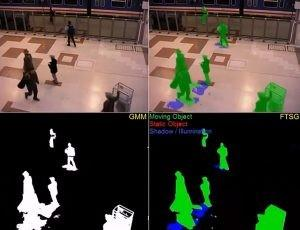Figure 4. Object recognition

## 5.jpg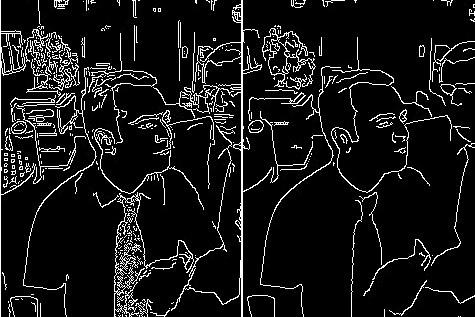Figure 5. Accurate object detection

The time complexity of the proposed and existing methods are depicted in Figure 6. The time complexity of the proposed method is low when compared to the traditional methods.

## 6.jpgFigure 6. Time complexity levels

CNN translated its good compute complexity in the execution time as weighted different values respectively. Thus obtaining more resources has multimedia task, resulting in increased complexity.

The True Positive Rate (TPR) and False Positive Rate (FPR) on the considered datasets is compared with the existing datasets and the parameter values are represented in Table 2.

Table 2. TPR and FPR values

 Features TPR FPR No Filter Filtered No Filter Filtered YOLO 96.23 97.25 11.5 15.8 MSRD 94 93.6 12.8 16.2 MSER+SURF 92.35 90.18 16.9 18.7 SURF+SIFT 89.55 85.50 20.5 15.8

## 7.png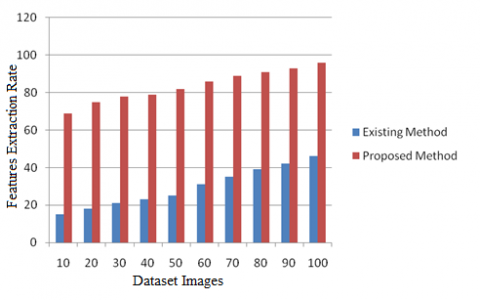Figure 7. Feature extraction rate

The Feature Extraction Rate is depicted in Figure 7 that represents that the proposed method extracts more features when compared to traditional method.

The accuracy Levels of the proposed and existing methods are depicted in Figure 8. The proposed method exhibits better accuracy rate than the existing method.

## 8.png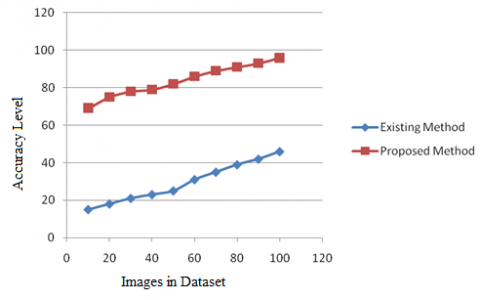Figure 8. Accuracy levels in feature extraction

5. Conclusions

Handling Big data now a days is a challenging task and extraction of objects from the video is having much importance for providing security. The data separated from the procedure of broad information mining significantly reduces computational expenses and in the same way it expands the adequacy and effectiveness. It turns out to be computationally conceivable to find the model information from huge measured unstructured information likewise by utilizing fitting mining techniques. Feature extraction is used for extracting relevant important features in computerized images in combination with clustering. Feature extraction could reveal coordinates among various regions in an image which could demonstrate the proximity of features. The method of reasoning behind utilizing clustering is that we have to recognize unique matches and features for example coordinates among unique regions of image. Convolutional Neural Network is effectively been applied to the field of image characterization. The CNN is mainly used for extracting hidden features in images for accurate object detections in the image or in a video. The proposed work achieves 96% accuracy in identifying of objects from Big data images. In future the features can be accurately defined such that the object with exact edges can be recognized.

References

 Ahmadi, S. (2019). A rule-based Kurdish text transliteration system. ACM Transactions on Asian and Low-Resource Language Information Processing, 18(2): 1-8. https://doi.org/10.1145/3278623

 Smirnova, A., Cudré-Mauroux, P. (2018). Relation extraction using distant supervision: A survey. ACM Computing Surveys, 51(5): 1-35. https://doi.org/10.1145/3241741

 Liu, X., Song, Q., Zhang, P.Z. (2018). Relation extraction based on deep learning. IEEE/ACIS 17th International Conference on Computer and Information Science (ICIS), 1: 687-691. 10.1109/ICIS.2018.8466437

 Singhal, A., Simmons, M., Lu, Z. (2016). Text mining for precision medicine: Automating disease-mutation relationship extraction from biomedical literature. Journal of the American Medical Informatics Association, 23(4): 766-772. https://doi.org/10.1093/jamia/ocw041

 Zhou, G.D., Qian, L.H., Fan, J.X. (2010). Tree kernel-based semantic relation extraction with rich syntactic and semantic information. Information Sciences, 180(8): 1313-1325. https://doi.org/10.1016/j.ins.2009.12.006

 Oramas, S., Espinosa-Anke, L., Sordo, M., Saggion, H., Serra, X. (2016). Information extraction for knowledge base construction in the music domain. Data & Knowledge Engineering, 106: 70-83. https://doi.org/10.1016/j.datak.2016.06.001

 Ryu, P.M., Jang, M.G., Kim, H.K. (2014). Open domain question answering using Wikipedia-based knowledge model. Information Processing & Management, 50(5): 683-692. https://doi.org/10.1016/j.ipm.2014.04.007

 Berndt, D.J., McCart, J.A., Finch, D.K., Luther, S.L. (2015). A case study of data quality in text mining clinical progress notes. ACM Transactions on Management Information Systems, 6(1): 1-21. https://doi.org/10.1145/2669368

 Faustina Joan, S.P., Valli, S. (2019). A survey on text information extraction from born-digital and scene text images. Proceedings of the National Academy of Sciences, India Section A: Physical Sciences, 89(1): 77-101. https://doi.org/10.1007/S40010-017-0478-Y

 Hogenboom, F., Frasincar, F., Kaymak, U., de Jong, F. (2011). An overview of event extraction from text. In Workshop on Detection, Representation, and Exploitation of Events in the Semantic Web (DeRiVE 2011) at Tenth International Semantic Web Conference (ISWC 2011), pp. 48-57.

 Song, Y.Q., Li, Y. (2012). Image Mining by data compactness and manifold learning. In Proceedings of the Fifth International Conference on Intelligent Networks and Intelligent Systems, Tianjin, China, pp. 29-32. https://doi:10.1109/ICINIS.2012.53

 Maddumala, V.R., Arunkumar, R. (2020). Big data-driven feature extraction and clustering based on statistical methods. Traitement du Signal, 37(3): 387-394. https://doi.org/10.18280/ts.370305

 Sazali, S.S., Rahman, N.A., Bakar, Z.A. (2016). Information extraction: Evaluating named entity recognition from classical Malay documents. In 2016 Third International Conference on Information Retrieval and Knowledge Management (CAMP), Bandar Hilir, Malaysia, pp. 48-53. https://doi.org/10.1109/INFRKM.2016.7806333

 Jung, K., Kim, K.I., Jain, A.K. (2004). Text information extraction in images and video: A survey. Pattern Recognition, 37(5): 977-997. https://doi.org/10.1016/j.patcog.2003.10.012

 You, Q.Z., Cao, L.L., Cong, Y., Zhang, X.C., Luo, J.B. (2015). A multifaceted approach to social multimedia-based prediction of elections. IEEE Transactions on Multimedia, 17(12): 2271-2280. http://doi:10.1109/TMM.2015.2487863

 Wang, C.B., Ma, X.G., Chen, J.G., Chen, J.W. (2018). Information extraction and knowledge graph construction from geoscience literature. Computers & Geosciences, 112: 112-120. https://doi.org/10.1016/j.cageo.2017.12.007

 Reyes, O., Ventura, S. (2018). Evolutionary strategy to perform batch-mode active learning on multi-label data. ACM Transactions on Intelligent Systems and Technology, 9(4): 1-26. https://doi.org/10.1145/3161606

 Mukund, S., Srihari, R., Peterson, E. (2010). An information-extraction system for Urdu---a resource-poor language. ACM Transactions on Asian Language Information Processing, 9(4): 1-43. https://doi.org/10.1145/1838751.1838754

 Nuray-Turan, R., Kalashnikov, D.V., Mehrotra, S. (2013). Adaptive connection strength models for relationship-based entity resolution. Journal of Data and Information Quality, 4(2): 1-22. https://doi.org/10.1145/2435221.2435224

 Zhang, Z.Q., Gao, J., Ciravegna, F. (2018). SemRe-rank: Improving automatic term extraction by incorporating semantic relatedness with personalized pagerank. ACM TKDD, 12(5): 1-41.

 Adrian, W.T., Leone, N., Manna, M., Marte, C. (2017). Document layout analysis for semantic information extraction. AI*IA 2017 Advances in Artificial Intelligence. AI*IA 2017. Lecture Notes in Computer Science, vol. 10640. Springer, Cham. https://doi.org/10.1007/978-3-319-70169-1_20

 Zhang, H.G., Zhao, K.L., Song, Y.Z., Guo, J. (2019). Text extraction from natural scene image: A survey. Neurocomputing, 122: 310-323. https://doi.org/10.1007/s11704-015-4488-0